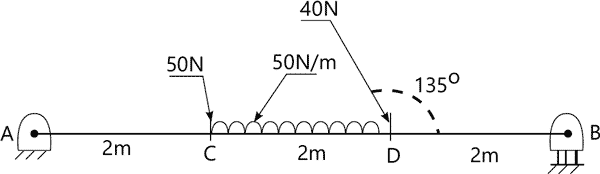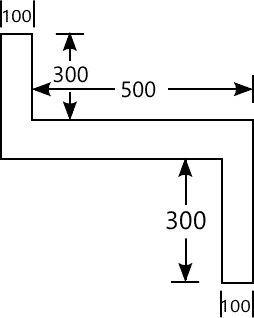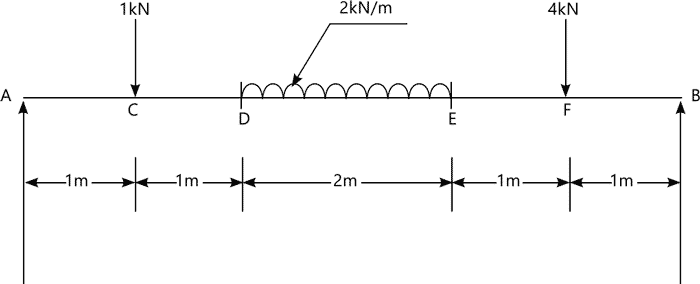MORE IN Basic Civil Engg. & Engg. Mechanics
RGPV First Year Engineering (Set B) (Semester 1)
Basic Civil Engg. & Engg. Mechanics
May 2013
Total marks: --
Total time: --
INSTRUCTIONS
(1) Assume appropriate data and state your reasons
(2) Marks are given to the right of every question
(3) Draw neat diagrams wherever necessary

Answer any one question from Q1 & Q2
1 (a) What do you understand by lab test and Field Test? Describe various field tests applied on bricks to judge the quality of bricks.
7 M
1 (b) Differentiate between the following:
i) Plastering and pointing ii) Concrete and mortar.
7 M

2 (a) Define the term workability. Discuss various factors which affect workability of concrete.
7 M
2 (b) Differentiate between the following:
i) Footing and Foundation ii) Bleeding and segregation
7 M

Answer any one question from Q3 & Q4
3 (a) State various methods of plane Table surveying. Explain any one method in detail.
7 M
3 (b) A 20m chain was used to measure a distance of 8000m. After measuring 3500m. It was found that the chain was 3cm too long which was correct in the beginning of the work. Again it was found at the end of the work that chain was 6cm too long. Calculate the correct distance measured
7 M

4 (a) Explain the following:
i) EDM method. ii) Reciprocal levelling.
6 M
4 (b) The following consecutive readings were taken with a level and a 4.0m staff on a continuously sloping ground at common interval of 30m. 0.780, 1.535, 1.955, 2.430, 2.985, 3.480, 1.155, 1.960, 2.365, 3.640, 0.935, 1.045, 1.630 and 2.545. The reduced level of the first point 'A' was 180.750 m. Rule out a page of a level field book and enter the above readings. Calculate the reduced levels of all the points and apply proper check for calculation.
8 M

Answer any one question from Q5 & Q6
5 (a) What are the various direct and indirect methods of contouring? Explain any one indirect method of contouring
7 M
5 (b) A railway embankment is 12m wide. The ground is level in the direction transverse to the centre line. Calculate the volume contained in a 100m length by Trapezoidal and prismoidal rule, if the side slope is 1:5:1. The centre height at 20m interval are 3.7m, 2.6m, 4.0m, 3.4m, 2.8m, 3.0m, 2.2m.
7 M

6 (a) Define the following terms related to contour
i) Contour
ii) Horizontal equivalent
iii) Cliff
iv) Contour Interval
vi) Ghat Tracer
6 M
6 (b) What do you understand by survey stations? Discuss requirements of a good survey station.
8 M

Answer any one question from Q7 & Q8
7 (a) Explain the following in detail.
i) Varignon's theorem ii) Conditions of equilibrium
7 M
7 (b) A machine component of length 2.5m and height 1m is carried upstairs by two men, who hold it by the front and back edges of its lower face. If the machine component is inclined at 30°to the horizontal and weights 100N, find how much of the weight each man shares.
7 M

8 (a) What is couple? Differentiate between moment and couple.
7 M
8 (b) A beam AB of 6m span is loaded as shown in Fig. below. Determine the reactions at A & B.7 M

Answer any one question from Q9 & Q10
9 (a) State and prove the theorem of parallel axis.
7 M
9 (b) Determine the principle moment of inertia of the section show in fig. below about its centroid. All dimensions are in mm.7 M

10 (a) Show with the help of neat sketches the various types of beams and loadings.
6 M
10 (b) Draw the shear force and bending moment diagram for the beam loaded as shown in fig. below. Clearly mark the position of maximum bending moment and determine its value.8 M

More question papers from Basic Civil Engg. & Engg. Mechanics